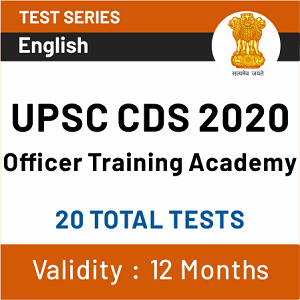# CDS Syllabus 2020: Check CDS Detailed Syllabus and Exam Pattern

CDS Syllabus 2020: The “Combined Defence Services” (CDS) Examination is conducted twice a year by the Union Public Service Commission for recruitment into the Indian Military Academy, Officers Training Academy, Indian Naval Academy and Indian Air Force Academy. The Notification for the examination is usually released in the months of October/November and June/July, and the examinations are conducted in February and November respectively. Only unmarried graduates are eligible to sit for the exam. Successful candidates are admitted into the respective Academies after an interview conducted by the Services Selection Board (SSB). To clear the examination, first, you need is the complete information of the pattern, syllabus and previous year exam analysis so that it would become easy for you to analyse the examination and to prepare a strategy further.

## CDS Syllabus 2020: Overview

 Mode of exam Offline Number of sections Indian Military Academy (IMA), Indian Naval Academy (INA) and Air Force Academy (AFA) – Three Officers’ Training Academy (OTA) – Two Total marks of the test IMA, INA and AFA – 300 OTA – 200 Nature of questions Objective type Language of question paper Hindi and English Duration of the test 2 hours for each section of test

## CDS Syllabus 2020: Exam Pattern for IMA, INA, AFA

Subject Duration Maximum marks
English 2 hours 100
General Knowledge 2 hours 100
Elementary Mathematics 2 hours 100## CDS Syllabus 2020: Exam Pattern for OTA

Subject Duration Maximum marks
English 2 hours 100
General Knowledge 2 hours 100

## CDS Syllabus 2020: Marking Scheme

Negative marking is applicable for every wrong marked answer. In CDS paper, each question carries 4 options and out of which only 1 option is correct. For each incorrect answer, 1/3 of the marks will be deducted. More than one option marked is treated as incorrect. However, if a question is left unanswered there will be no negative marking.

## CDS Syllabus 2020: Subject-Wise Syllabus

Candidates can check the UPSC CDS Syllabus in the table given below.

Sections Topics
English
• Spotting Errors Questions
• Sentence Arrangement Questions
• Synonyms & Antonyms
• Selecting Words
• Ordering of Sentence
• Comprehension Questions
• Ordering of words in a sentence
• Fill in the blanks questions
• Idioms and Phrases
General Awareness
• Economics
• Physics
• Current Awareness
• Politics
• Chemistry
• Sociology
• History
• Defence related Award
• Geography
• Environment
• Sport
• Biology
• Cultural
• Book
• Statement
• true/false
Elementary Mathematics Algebra
• Basic Operations
• simple factors
• Remainder Theorem
• H.C.F.
• L.C.M.
• Theory of polynomials
• relation between its roots and coefficients (Only real roots to be considered)
• Simultaneous linear equations in two unknowns—analytical and graphical solutions
• Simultaneous linear inequations in two variables and their solutions
• Practical problems leading to two simultaneous linear equations or inequations in two variables or quadratic equations in one variable & their solutions
• Set language and set notation
• Rational expressions and conditional identities
• Laws of indices
Arithematic
• Number System: Natural numbers, Integers, Rational and Real numbers.
• Fundamental operations: addition, substraction, multiplication, division, Square roots, Decimal fractions
• Unitary method
• time and distance
• time and work
• percentages
• applications to simple and compound interest
• profit and loss
• ratio and proportion
• variation
• Elementary Number Theory: Division algorithm
• Prime and composite numbers
• Tests of divisibility by 2, 3, 4, 5, 9 and 11
• Multiples and factors. Factorisation Theorem
• H.C.F. and L.C.M.
• Euclidean algorithm
• Logarithms to base 10
• laws of logarithms
• use of logarithmic tables
Trigonometry
• Sine ×, cosine ×, Tangent × when 0° < × < 90°
• Values of sin ×, cos × and tan ×, for ×= 0°, 30°, 45°, 60° and 90°
• Simple trigonometric identities
• Use of trigonometric tables
• Simple cases of heights and distances
Geometry
• Lines and angles
• Plane and plane figures
• Theorems on Properties of angles at a point
• Parallel lines
• Sides and angles of a triangle
• Congruency of triangles
• Similar triangles
• Concurrence of medians and altitudes
• Properties of angles
• sides and diagonals of a Parallelogram
• rectangle and square
• Circles and its properties including tangents and normals
• Loci
Mensuration
• Areas of squares
• rectangles
• parallelograms
• triangle and circle
• Areas of figures which can be split up into these figures (Field Book)
• Surface area and volume of cuboids
• lateral surface and volume of right circular cones and cylinders
• surface area and volume of spheres
Statistics
• Collection and tabulation of statistical data
• Graphical representation frequency polygons
• histograms
• bar charts
• pie charts etc
• Measures of central tendency

## CDS Syllabus 2020: FAQs

Q 1. How many stages CDS exam have?
Ans. There are only two stages, written exam and SSB exam
Q 2. Does CDS OTA and CDS IMA have different exam?
Ans. Yes, OTA and IMA have different exam with different sections.
Q 3. Is there any negative marking in the CDS?
Ans. Yes, there is a negative marking in prelims of 1/3 marks.
Q 4. How many sections CDS IMA paper have?
Ans. There are 3 sections in CDS IMA paper.
Q 5. How many sections CDS OTA paper have?
Ans. There are only 2 sections in CDS OTA paper.

## Join the conversation

1.2.1.Kiran says:
2.Vivek says: